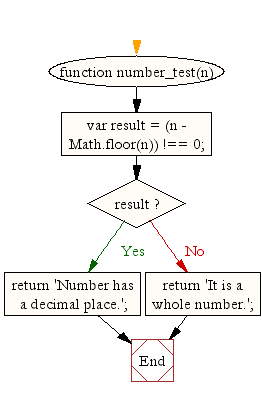# JavaScript: Check if a number is a whole number or has a decimal place

## JavaScript Math: Exercihse-38 with Solution

Write a JavaScript function to check if a number is a whole number or has a decimal place.

Note : Whole Numbers are simply the numbers 0, 1, 2, 3, 4, 5, ... (and so on). No Fractions!
Test Data :
console.log(number_test(25.66));
"Number has a decimal place."
console.log(number_test(10));
"It is a whole number."

Pictorial Presentation:Sample Solution:-

HTML Code:

``````<!DOCTYPE html>
<html>
<meta charset="utf-8">
<title>JavaScript function to check if a number is a whole number or has a decimal place</title>
<body>

</body>
</html>
```
```

JavaScript Code:

``````function number_test(n)
{
var result = (n - Math.floor(n)) !== 0;

if (result)
return 'Number has a decimal place.';
else
return 'It is a whole number.';
}

console.log(number_test(25.66));

console.log(number_test(10));
```
```

Sample Output:

```Number has a decimal place.
It is a whole number.
```

Flowchart:Live Demo:

See the Pen javascript-math-exercise-38 by w3resource (@w3resource) on CodePen.

Improve this sample solution and post your code through Disqus

What is the difficulty level of this exercise?

Test your Programming skills with w3resource's quiz.

﻿

## JavaScript: Tips of the Day

data storage

```sessionStorage.setItem('cool_secret', 123);
```

The data stored in sessionStorage is removed after closing the tab.
If you used localStorage, the data would've been there forever, unless for example localStorage.clear() is invoked.

Ref: https://bit.ly/323Y0P6